btvcvar
Estimate a Bayesian time-varying coefficients VAR, or BTVCVAR, model.
Syntax
var_name.btvcvar(options) lag_pairs endog_list [@ exog_list]
btvcvar estimates a Bayesian time-varying coefficients VAR. The order of the VAR is specified using a lag pair, followed by a list of series or groups for endogenous variables. Exogenous variables can be included using the @-sign followed by a list of series or groups. A constant is automatically added to the list of exogenous variables; to estimate a specification without a constant, use the noconst option.
Options
Prior hyper-parameters

 T0 = int(default = 0) Set prior sample size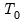. A prior sample is not used if T0 is set to 0. To use a prior sample, T0 must be set to an integer larger than the number of coefficients per equation in a standard VAR version of the model. tau0 = num(default = 5.0) Set prior scaling parameter for the initial state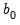. tau1 = num(default = 1.0) Set prior scaling parameter for the observation covariance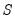. nu1 = num(default = 5.0) Set prior dof parameter for the observation covariance. tau2 = num(default = 0.01) Set prior scaling parameter for the process covariance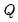. nu2 = num(default = 5.0) Set prior dof parameter for the process covariance.
Display options

 usemean Use posterior mean as the point estimate. The posterior median is used if usemean is not included in the options list. showci Show credibility intervals (bands). cilevels = arg(default = "0.95") Set credibility levels. For multiple levels, enter a space-delimited list of values surrounded by quotation marks, e.g., "0.3 0.5 0.8". uselines Use lines instead of shading for credibility intervals.
MCMC options

 burn = int(default = 5000) Set burn-in size. size = int(default = 5000) Set posterior sample size. thin = int(default = 1) Set thinning size. A thinning size of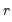indicates that every-th draw after the burn-in period is stored. nsub = int Set the number of subchains. seed = int Set the random seed. EViews will generate a seed if one is not specified. rng = arg(default = “kn” or method set via rndseed) Set random number generator type. Available types are: improved Knuth generator (“kn”), improved Mersenne Twister (“mt”), Knuth’s (1997) lagged Fibonacci generator used in EViews 4 (“kn4”), L’Ecuyer’s (1999) combined multiple recursive generator (“le”), Matsumoto and Nishimura’s (1998) Mersenne Twister used in EViews 4 (“mt4”).
Other options

 smoother = arg(default = "CFA") Set simulation smoothing method. Available methods are "CFA" (Cholesky factor algorithm), "KFS" (Kalman filter and smoother), and "MMP" (McCausland, Miller, & Pelletier, 2011). stable Use the method of Cogley & Sargent for obtaining stable draws. maxattempts = int(default = 100) Set the maximum number of attempts for the sampler to draw stable VAR coefficients for all dates in the data sample. noconst Do not include a constant in the exogenous regressors list.
Examples
To declare and estimate a BTVCVAR named MYVAR with endogenous variables DGP and UNEMP, a constant, the first lag, and a prior sample of size 40, run
var myvar.btvcvar(t0=40) 1 1 gdp unemp
in the command window. Running the command
var myvar.btvcvar(t0=40, showci, cilevels="0.3 0.5") 1 1 gdp unemp
will also display shaded 30% and 50% credibility bands. For reproducible results, also set the number of subchains (nsub), the random seed (seed), and the random number generator type (rng):
var myvar.btvcvar(t0=40, nsub=12, seed=342458900, rng=kn, showci, cilevels="0.3 0.5") 1 1 gdp unemp
Command capture will always show the nsub, seed, and rng options.
Cross-references
See “Bayesian Time-varying Coefficients VAR Models” for details.